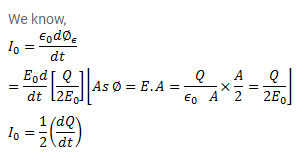# Consider the situation of the previous problem.

Question:

Consider the situation of the previous problem. Define displacement resistance $R_{d}=V / i_{d}$ of the space between the plates where $\mathrm{V}$ is the potential difference between the plates and $\mathrm{i}_{\mathrm{d}}$ is the displacement current. Show that $\mathrm{R}_{\mathrm{d}}$ varies with time as $R_{d}=R\left(e^{1 / r}-1\right)$.

Solution: